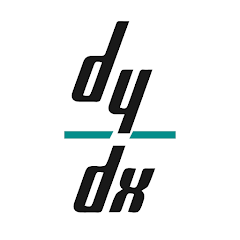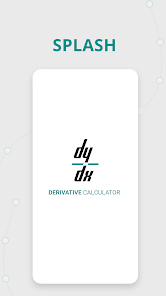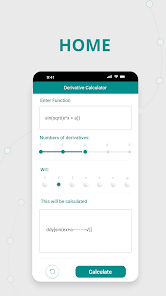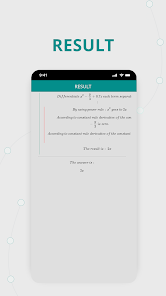# Derivative Calculator

10K+EveryoneDerivative Calculator enables you to compute math functions through differentiation. This Derivative Solver App gives you detailed step by step solution of derivation. So, you can easily understand the implementation of derivative formula of dy/dx.

Derivative Solution App helps to quickly solve the calculation of derivatives. You don’t need to do manual calculation of Derivation, when you have this derivative equation calculator app. Insert the question with correct values, and hit the calculate button. Get accurate solutions with graph and plots by using this Derivative Calculator with Steps.

We have made this differentiation calculator with the latest techniques to Solve Derivatives. That is why it saves a lot of your time. You can solve your assignment and tough equations of Deriv. If you lack time and looking for a good Derivative Solution App! Try this Free Derivative Calculator and get accurate results.

## Benefits of Math Derivative Solver

It is simple to use a derivative calculus calculator. Here are some simple steps for teachers and students of calculus. So that everyone can easily use this Derivative Calculator step by step.

Beginners: By using the differential calculator to solve the function, any beginner to differentiation can have some practice which will later save them in their tests and exams season.

Senior students: Those who already understand derivatives, can still use this derivation solver. They can use it to solve assignments or learn to evaluate any tough question of Derive.

Teachers: Yes, even teachers will find this derivative solution app helpful. It can be used to check assignments, when you hit a dead end while differentiating by using this dy/dx calculator.

Anyone up for learning: Those who are not a student or teaching in some institute, but just want to learn derivatives, because they find them interesting (yeah many people love math). They can easily learn the Derivative Formula and its implementation with this calculus calculator with steps.
Similarly, math article writers can solve examples of derivative calculation here that they want to add to their posts.

## Features of Derivative Calculator

- Good for students and teachers of Calculus.
- Implementation of formula with steps.
- Smooth working derivative solver.
- Supports almost all devices.
- Very lightweight Derivation Calculator App.
- Quick derivative calculation with graph and plots.
- Easy to find the Derivative of a function.

Multiple Differentiation
You can differentiate the same function for first, second, third, fourth, and fifth Derivatives. All you have to do is enter the number in the labeled box of Derivative.

Step by Step Solution
Derivative Differentiation Calculator gives you the complete solution which contains all the steps of the Derivation. It helps to understand derivatives very much.

Example Function
This Derivative Calculator can be used by any newbie. The example functions can give them basic know-how in that regard to understand the solution process of Calculus Derivatives.

Plots
One plus point of using this Derivative Calculator with Steps is that, you get a plot for almost every solved function.

These were some of the many features, which help you to easily and quickly solve the complex equations of derive. This Math Derivative Solver will be your favorite app to solve derivatives, after using it once.

We sure that you’ll like this Derivative Calculator. It is simple to use and provides accurate solutions for derivatives with steps. We would love to hear your views & suggestions about this Calculus Derivatives Calculator. So that, we’ll be able to improve the Derivative calculation as per your suggestions and make it a wonderful and excellent working Derivative Solution App.
Updated on
Sep 12, 2023

## Data safety

Safety starts with understanding how developers collect and share your data. Data privacy and security practices may vary based on your use, region, and age. The developer provided this information and may update it over time.No data shared with third parties